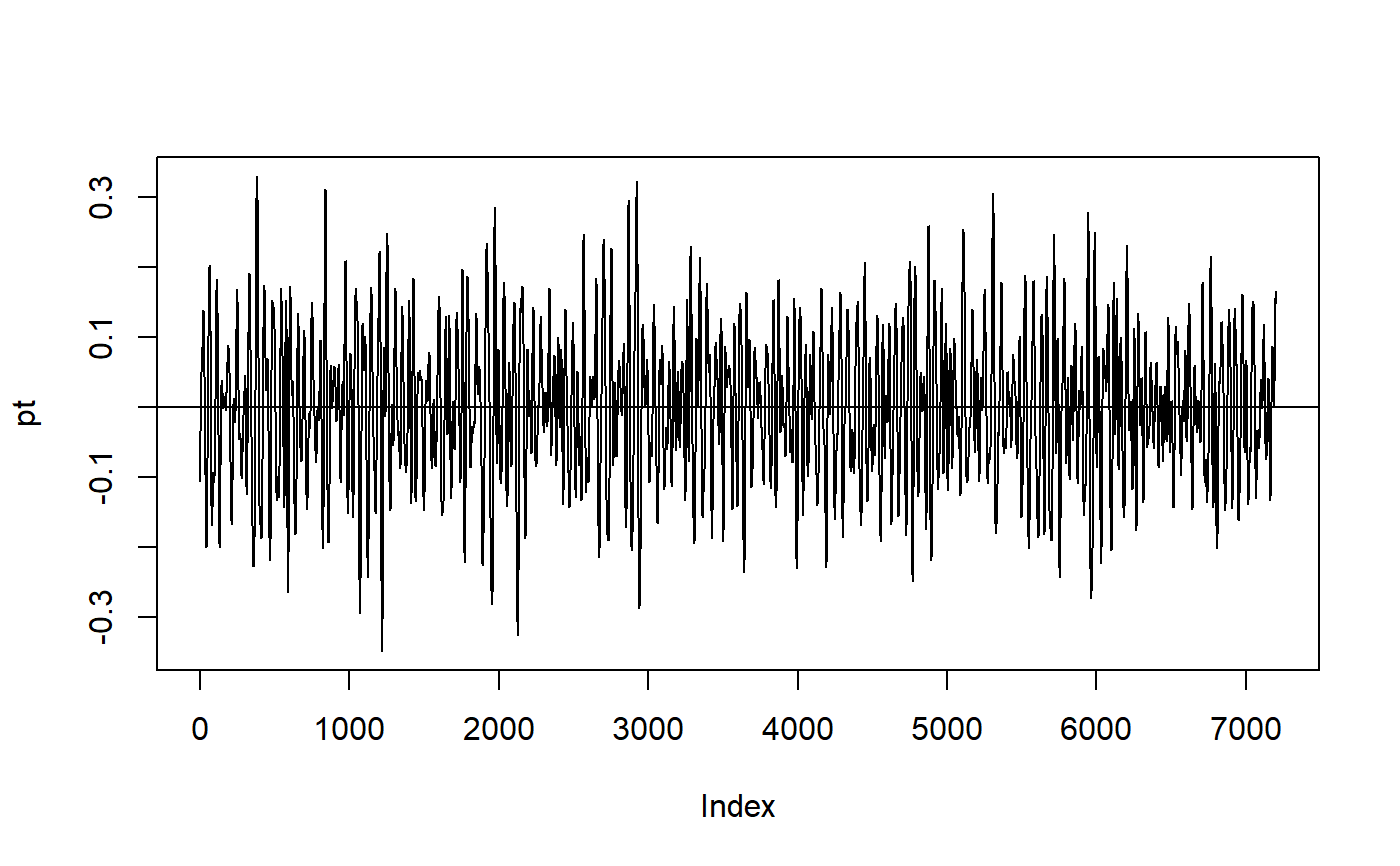Fits a straight line to a vector of values using lm(), and uses the regression coefficients to subtract off the linear trend from the values.

detrendHeight(pt)

## Arguments

pt A vector of numeric values to be detrended

## Value

A list containing the following:

pt A vector of detrended values

seg_len The segment length used

h The mean height of the water column

trend A two element vector of the intercept and slope from the linear regression.

## Details

Typically this is used to remove a tidal trend from a ocean surface height time series before attempting to calculate statistics for waves, which are variations above and below the mean surface height. Returns a series of residuals around the linear trend, in the original units of measurement (typically meters).

## Examples

data(wavedata)
detrended <- detrendHeight(wavedata\$swDepth.m)
pt <- detrended[['pt']]
plot(pt, type = 'l')abline(h = 0)